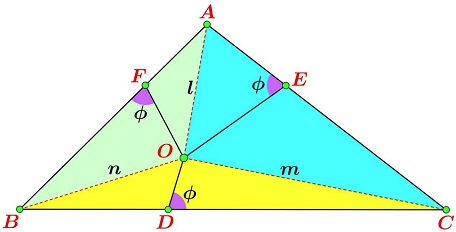# Generalized Carnot's theorem

Carnot's theorem is a direct application of the theorem of Pythagoras. It's a nice little theorem that is unlikely to be rediscovered with dynamic geometry software. However, Miguel Ochoa Sanchez (Peru) has come up with an interesting generalization.

### Miguel Ochoa Sanchez

19 December 2015

Point $O$ is within $\Delta ABC.\;$ Let points $D,$ $E,$ and $F$ be located on the sides $BC,$ $AC,$ and, respectively, $AB$ of the triangle such that the three angles $AEO,$ $BFO,$ and $CDO$ are all equal to, say, $\phi.$Then

$AF^{2} - BF^{2} + BD^{2} - CD^{2} + CE^{2} - AE^{2} = 4[\Delta ABC]\cdot\cot\phi,$

where $[\Delta ABC]$ is the area of $\Delta ABC].$

The proof depends on the following

### Lemma

Let $D$ be on the side of $BC$ of $\Delta ABC;$ $\angle ADC=\phi.$Then

$CD^{2} - BD^{2} = AC^{2} - AB^{2} + 4[\Delta ABC]\cdot\cot\phi,$

### Proof of Lemma

Drop the perpendicular $AH$ to $BC.$Then $DH=AH\cdot\cot\phi,$ $CH=CD-AH\cdot\cot\phi.$ By the Pythagorean theorem,

\begin{align} AC^{2}-AB^{2}&=CH^{2}-BH^{2}\\ &=(CD-AH\cdot\cot\phi)^{2}-(BD+AH\cdot\cot\phi)^{2}\\ &=CD^{2}-BD^{2}-2\cdot CD\cdot AH\cdot\cot\phi-2\cdot BD\cdot AH\cdot\cot\phi\\ &=CD^{2}-BD^{2}-2\cdot BC\cdot AH\cdot\cot\phi\\ &=CD^{2}-BD^{2}-4\cdot[\Delta AB]\cdot\cot\phi. \end{align}

### Proof of Theorem

We'll use the notations as in the diagram below:Apply the lemma to triangles $BOC,$ $AOB,$ $AOC$ to obtain

\begin{align} CD^{2}-BD^{2}&=m^{2}-n^{2}+4[\Delta BOC]\cot\phi\\ BF^{2}-AF^{2}&=n^{2}-l^{2}+4[\Delta BOC]\cot\phi\\ AE^{2}-CE^{2}&=l^{2}-m^{2}+4[\Delta BOC]\cot\phi. \end{align}

\begin{align} CD^{2}-BD^{2}&+BF^{2}-AF^{2}+AE^{2}-CE^{2}\\ &=4[\Delta BOC]\cot\phi+4[\Delta BOC]\cot\phi+4[\Delta BOC]\cot\phi\\ &=4[\Delta ABC]\cot\phi. \end{align}

There is a natural generalization to the case of an $n\text{-gon},$ with $n\ge 3:$

With $P_{n+1}=P_1,$

$\displaystyle\sum_{k=1}^{n}P_iQ_i^2-\sum_{k=1}^{n}Q_iP_{i+1}^2=4[P_1P_2\ldots P_n]\cot\phi.$The proof is analoguous to the case where $n=3.$